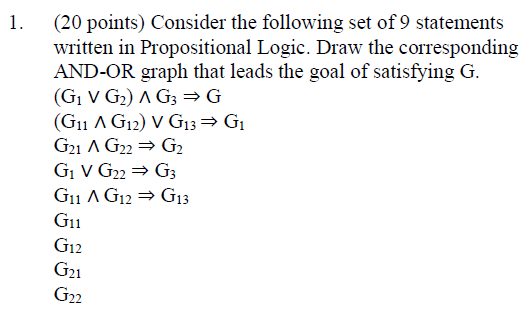Home / Expert Answers / Computer Science / 20-points-consider-the-following-set-of-9-statements-written-in-propositional-logic-draw-the-co-pa820

# (Solved): (20 points) Consider the following set of 9 statements written in Propositional Logic. Draw the co ...(20 points) Consider the following set of 9 statements written in Propositional Logic. Draw the corresponding AND-OR graph that leads the goal of satisfying $$G$$. $\begin{array}{l} \left(\mathrm{G}_{1} \vee \mathrm{G}_{2}\right) \wedge \mathrm{G}_{3} \Rightarrow \mathrm{G} \\ \left(\mathrm{G}_{11} \wedge \mathrm{G}_{12}\right) \vee \mathrm{G}_{13} \Rightarrow \mathrm{G}_{1} \\ \mathrm{G}_{21} \wedge \mathrm{G}_{22} \Rightarrow \mathrm{G}_{2} \\ \mathrm{G}_{1} \vee \mathrm{G}_{22} \Rightarrow \mathrm{G}_{3} \\ \mathrm{G}_{11} \wedge \mathrm{G}_{12} \Rightarrow \mathrm{G}_{13} \\ \mathrm{G}_{11} \\ \mathrm{G}_{12} \\ \mathrm{G}_{21} \\ \mathrm{G}_{22} \end{array}$

We have an Answer from Expert